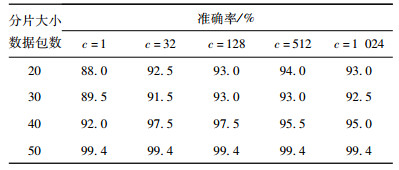# 基于云流量混淆的Tor匿名通信识别方法（二）4 实验评估4.1 实验环境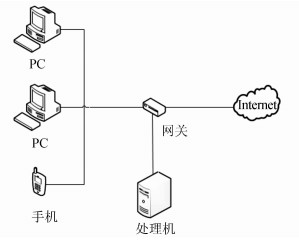4.2 流量识别的评价标准

 recall=TP/(TP+FN),accuracy=(TP+TN)/(TP+FN+FP+TN)。$\begin{array}{l}\phantom{\rule{thickmathspace}{0ex}}\phantom{\rule{thickmathspace}{0ex}}\phantom{\rule{thickmathspace}{0ex}}\phantom{\rule{thickmathspace}{0ex}}\phantom{\rule{thickmathspace}{0ex}}\phantom{\rule{thickmathspace}{0ex}}\phantom{\rule{thickmathspace}{0ex}}\phantom{\rule{thickmathspace}{0ex}}\phantom{\rule{thickmathspace}{0ex}}\phantom{\rule{thickmathspace}{0ex}}\phantom{\rule{thickmathspace}{0ex}}\phantom{\rule{thickmathspace}{0ex}}\phantom{\rule{thickmathspace}{0ex}}\phantom{\rule{thickmathspace}{0ex}}\phantom{\rule{thickmathspace}{0ex}}\phantom{\rule{thickmathspace}{0ex}}\phantom{\rule{thickmathspace}{0ex}}recall=TP/\left(TP+FN\right),\\ accuracy=\left(TP+TN\right)/\left(TP+FN+FP+TN\right)。\end{array}$

4.3 基于特征匹配识别的实验评估

4.3.1 不同长度流的识别准确率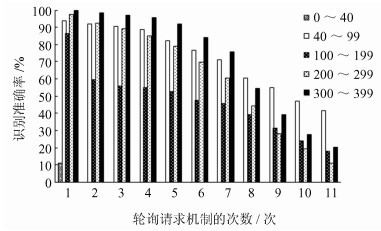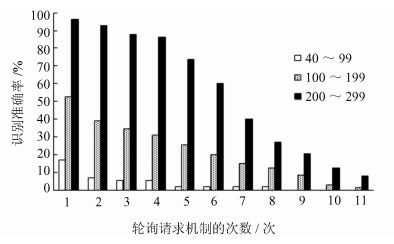4.3.2 不同Tor Browser版本的识别准确率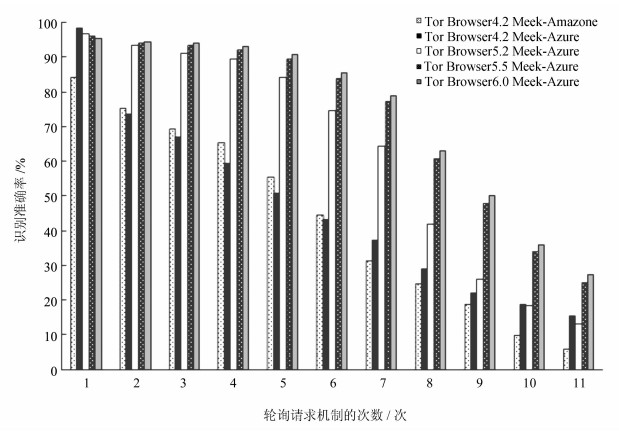4.3.3 基于特征匹配的识别准确率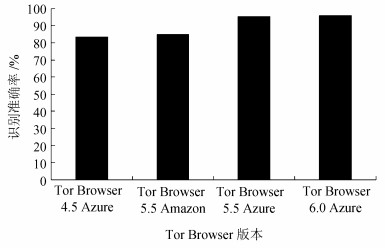4.3.4 实时性分析

4.4 基于SVM识别的实验评估

4.4.1 分片大小对识别准确率的影响